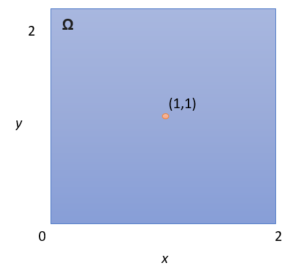# Continuous Sample Space: Simple Definition, Examples

Share on

Sample spaces introduced in early probability classes are typically discrete. That is, they are made up of a finite (fixed) amount of numbers. For example, if you roll a die, the sample space (Ω) is [1, 2, 3, 4, 5, 6]. Rolling a twenty-sided die or choosing a card from a deck all produce discrete sample spaces. A continuous sample space is based on the same principles, but it has an infinite number of items in the space. In other words, you can’t write out the space in the same way that you would write out the sample space for a die roll. With a continuous sample space, you would still be writing numbers long after the sun has imploded into a black hole.

## Continuous Sample Space Example

The following image shows a continuous sample space— an area 2 units high and 2 units wide. Imagine that the space represents a game where a dart is dropped into the space and lands on a random spot. What are the odds of the dart landing on the spot pictured?It may surprise you to learn that the odds of the ball landing on either of those two spots is zero. In fact, the odds of the dart falling in any spot at all equals zero. Let’s say the dart lands at (1, 1). It could also land a hair to the right, at (1.001, 1). Or, an even tinier fraction to the right, at (1.0000000001, 1). If you try and write down all of the possible places the dart could land, you won’t be able to, because there are an infinite number of spaces the ball could land on. If you have a problem with this logic, you aren’t alone. It’s an example of how we think the world works, and how it actually works. A famous example of how infinity messes with logic is Zeno’s paradox (also known as “&helli[;the hare can never overtake the tortoise in a race”).

A more modern approach is to use the problem in terms of limits. In simple terms, a limit is the number very close to the one you’re measuring. In the case of all these zeros (1.001, 1.0000001, 1. 00000000000001 etc.), you could say they all are very close to 1, giving you far fewer points to deal with. For the above problem, you could come up with a very reasonable probability if you could all the possible whole numbered coordinates: (0, 1), (0, 2), (0, 3) and so on.

## References

Bertsekas, D. & Tsitsiklis, J. (2008). Introduction to Probability. Athena Scientific.

CITE THIS AS:
Stephanie Glen. "Continuous Sample Space: Simple Definition, Examples" From StatisticsHowTo.com: Elementary Statistics for the rest of us! https://www.statisticshowto.com/continuous-sample-space/
---------------------------------------------------------------------------Need help with a homework or test question? With Chegg Study, you can get step-by-step solutions to your questions from an expert in the field. Your first 30 minutes with a Chegg tutor is free!